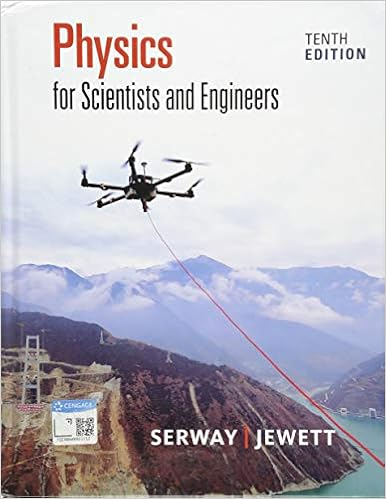# Lab 8 - Simple Harmonic Motion - LAB 8 Simple Harmonic...

• Lab Report
• 7
• 55% (11) 6 out of 11 people found this document helpful

This preview shows page 1 - 3 out of 7 pages.

##### We have textbook solutions for you!
The document you are viewing contains questions related to this textbook.The document you are viewing contains questions related to this textbook.
Chapter 15 / Exercise 13
Physics for Scientists and Engineers
Jewett/SerwayExpert Verified
8-1 LAB 8: Simple Harmonic Motion Hooke’s Law We all have some familiarity with springs . If we stretch a spring away from its relaxed (unstretched) length, if exerts a pulling force to try to return to its shorter state. Likewise, if we compress the spring, it exerts a pushing force to try to return to its lengthened state. This is called a restoring force: a force opposing the change to restore the system to its relaxed state. For most springs, the relationship between restoring force and displacement from the relaxed state is linear. For example, if we double the stretching (or compression), the force doubles. This relationship is called Hooke’s Law , and any spring obeying it is called an ideal spring. Eqn. 8-1 Hooke’s Law The negative sign is strictly a theoretical consideration to make the force vector in the opposite direction of the displacement. In free body diagrams you only need to worry about the magnitude (because you draw the vector in the correct direction). The coefficient k is called the spring constant and represents the spring’s stiffness. A stiffer sp ring resists displacement more, so it has a higher k. Consider the mass-spring system to the right. If it is in equilibrium , the forces on it are balanced with equal magnitudes but opposite directions. The downward force is of course just the weight of the hanging mass. The spring counters this with its restoring force. Hooke’s Law depends on us assuming the starting position for displacement measurements is at x = 0. If it is not, that’s okay. We simply need to say the displacement is the difference in position and modify Hooke’s Law as ⃑⃑⃑⃑⃑⃑⃑⃑⃑⃑⃑⃑⃑⃑⃑⃑⃑⃑⃑⃑ . Analyzing the free body diagram with a = 0, Spring Potential Energy Because we’re applying a force against the restoring force through some displacement distance , we’re doing work on the spring. That means we’re storing potential energy into it. Eqn. (8-2) Spring Potential Energy Real Springs An ideal spring obeys Hooke’s Law linearly. A real spring is not as perfect. Most springs can be called ideal because they obey Hooke’s Law for small displacements. At some point the approximation “breaks down” if it stretches too much. In these regions the spring may permanently deform or the metal ma y weaken. Other systems obeying Hooke’s Law (pendula, mostly) are linear for small angles, but become very different if they’re displaced too far.
##### We have textbook solutions for you!
The document you are viewing contains questions related to this textbook.The document you are viewing contains questions related to this textbook.
Chapter 15 / Exercise 13
Physics for Scientists and Engineers
Jewett/SerwayExpert Verified
8-2 Simple Harmonic Motion Any object that obeys Hooke’s Law will undergo oscillation if disturbed from its equilibrium position. As long as the restoring force is linear, the resulting oscillation is called simple harmonic motion . The object must be excited to oscillate by giving it some energy. It can either be given kinetic energy at its equilibrium position by striking it, or it can be given potential energy by being displaced away from its equilibrium position.
•••